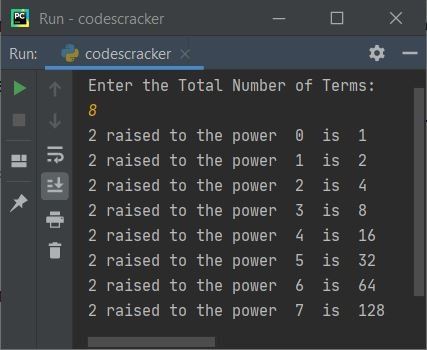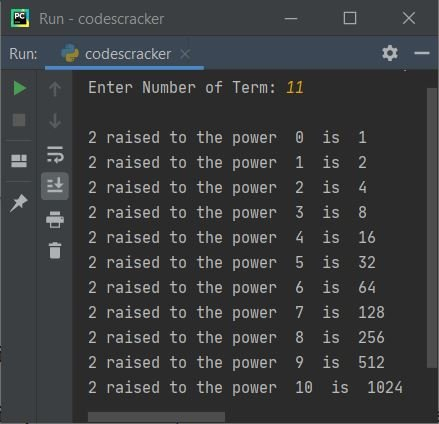# Python Program to Display Powers of 2

This article is created to cover some programs in Python, that find and prints powers of 2 upto any particular term defined by user at run-time. Here are the list approaches used:

• Simple Program to Display Powers of 2 upto N Terms. The value of N must be entered by user
• Display Powers of 2 using normal Function
• Using anonymous (lambda) Function

## Display Powers of 2 upto N Terms

To display powers of 2 upto given terms in Python, you have to ask from user to enter the total number of terms, then display powers of 2 from 0 to N-1 like shown in the program given below:

```print("Enter the Total Number of Terms: ")
tot = int(input())

for i in range(tot):
print("2 raised to the power ", i, " is ", 2 ** i)```

Here is the initial output produced by this Python program:Now supply the input say 8 and press `ENTER` key to find and print all powers of 2 upto 8 terms starting from 0 like shown in the snapshot given below:Note - The ** (exponentiation) operator in Python is used to find raised to power value. For example a ** b evaluated as ab. So 2 ** 3 returns 2*2*2, that is 8

If user enters input as 8, then 8 gets stored in tot. So from above program, the following code (after replacing the value of tot variable):

`for i in range(8):`

is created to execute the following statement (present inside its body):

`print("2 raised to the power ", i, " is ", 2 ** i)`

eight number of times with the value of i from 0 to 7 (8-1).

## Display Powers of 2 using Normal Function

This program does the same job as of previous program, but using a function named MyFun(). This function receives a value as its argument and returns 2 raised to the power of this value.

```def MyFun(x):
return 2 ** x

print("Enter Number of Term: ", end="")
tot = int(input())

print()
for i in range(tot):
print("2 raised to the power ", i, " is ", MyFun(i))```

Here is its sample run with user input 11 as total number of terms:The end= in above program, is used to skip inserting an automatic newline.

## Display Powers of 2 using Anonymous Function (lambda)

This is the last program of this article, created using an anonymous function. Let's have a look at the program first:

```print("Enter Number of Term: ", end="")
tot = int(input())

anoms = lambda x: 2 ** x
print()
for i in range(tot):
print("2 raised to the power ", i, " is ", anoms(i))```

This program produces same output as of previous program. In above program, the following statement:

`anoms = lambda x: 2 ** x`

states that, whatever we pass the value to anoms later on, the value gets copied to x and 2 ** x gets returned. That is, anoms(4) returns 2 ** 4 or 24 or 2*2*2*2 or 16.

Above program can also be approached directly in this way:

```print("Enter Number of Term: ", end="")
tot = int(input())

res = list(map(lambda x: 2 ** x, range(tot)))

print()
for i in range(tot):
print("2 raised to the power ", i, " is ", res[i])```

The list() is used to create a list. And the map() returns an iterator of results, after applying the function to each item of a given iterable (list in above program's case).

Python Online Test

« Previous Program Next Program »